# The smallest number

What is the smallest number that can be divided by both 5 and 7

Correct result:

x =  35

#### Solution: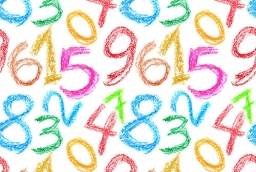We would be pleased if you find an error in the word problem, spelling mistakes, or inaccuracies and send it to us. Thank you!Tips to related online calculators
Do you want to calculate least common multiple two or more numbers?

## Next similar math problems:

• Lcm 2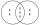Create the smallest possible number that is divisible by numbers 5,8,9,4,3
• Counting numberWhat is the smallest counting number divisible by 2,5,7,8 and 15?
• Unknown integerFind the smallest integer that: divided by 2, the remainder is 1 divided by 3 the remainder is 2, divided by 4 remainder is 3, ... divided by eight reminder is 7, by 9 reminder is 8.
• CherriesCherries in the bowl can be divided equally among 19 or 13 or 28 children. How many is the minimum cherries in the bowl?
• LCMWhat is the least common multiple of 5, 50, 14?
• LCM of two numberFind the smallest multiple of 63 and 147
• Square roomWhat is the size of the smallest square room, which can pave with tiles with dimensions 55 cm and 45 cm? How many such tiles is needed?
• LCMCommon multiple of three numbers is 3276. One number is in this number 63 times, second 7 times, third 9 times. What are the numbers?
• Length of a stringWhat is the smallest length of a string that we can cut into 18 equal parts and even 27 equal parts (in decimeters)?
• Lesson exercising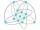The lesson of physical education, pupils are first divided into three groups so that each has the same number. The they redistributed, but into six groups. And again, it was the same number of children in each group. Finally they divided into nine equal g
• PlumsIn the bowl are plums. How many would be there if we can divide it equally among 8, 10 and 11 children?
• Lcm of three numbersWhat is the Lcm of 120 15 and 5
• FlowerbedsWhat is the smallest length of flowerbeds we have to prepare to plant seedlings at grid 20,30,25,40 cm?
• Lamps on playground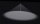The playground has the shape of a rectangle 36 x 50m. After how many meters will he place the lamps on his lighting, if the distances between them are to be the same on both sides if the builders want to use the smallest possible number of lamps?
• FractionsSort fractions ? by its size. Result write as three serial numbers 1,2,3.
• PexesoDrawing paper has dimensions of 60cm and 840mm. Pupils have to divide it into squares so that they can make a pexeso. What dimension must squares have if their side is to be larger than 3cm and less than 10cm?
• Dinosaurs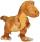More than 30 and less than 60 dinosaurs have met at the pond. A quarter of them bathed and 1/7 saws and the rest gripped. How many were at the pond? How many were there?